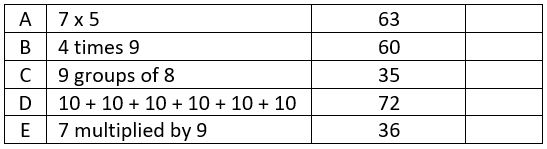## LetsPlayMaths.Com

WELCOME TO THE WORLD OF MATHEMATICS

### Class 2 - Half Yearly Examination – 1

Objective Question:              [2X10=20]

1. Which number comes after 899?

2. Which number is the greatest number from the below given numbers?

457, 978, 693, 989, 578.

3. Complete the given pattern.

245, 345, _____, _____, 645.

4. 60 + 45 – 23 = _____.

5. 689 – Smallest 3-digit number = _____.

6. 5 tens – 4 ones = _____.

7. _____ - 1 = 499.

8. The difference between successor and predecessor of any number is equal to ______.

9. 8, 16, 24, 32, _____.

10. If 10 is added 5 times, then the sum is ________.

Objective Question:              [4X5=20]

11. Form the greatest and smallest 3-digit number using the given digit only once.

9, 5, 3

12. From the below given numbers which one is odd, and which one is even numbers.

545, 982, 663, 72

13. In a train 872 people were travelling. 235 people got down in the first station and 23 people got down in the second station. How many people remained in the train?

14. In a school library there were 785 books. School bought 200 more books for the library. Same day students borrowed 430 books. How many books remained in the library?

15. Match the following: